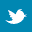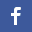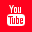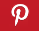Completely Solved C, C++ Programs Assignment.Quick Search Database Videos Tutorials Ebooks Forums FAQ Aboutus Household Industrial Manufacturing Service Shopping Transportation### Signal and System:Solved MATLAB Assignment II

 Filed Under: MATLAB,Signal and System

Signal and System:Solved MATLAB Assignment II
Problem 1:[A2P1] Let us consider y=sin(x). Plot y and dy/dx in the same window.
Problem 2: :[A2P2] Given a polynomial x4 – 12x3 + 0x2 + 25x +116, find the roots of it using Matlab. Also reconstruct, the polynomial from its roots.
Problem 3: :[A2P3] Generate and plot the following sequence over the indicated interval.
X(n) = 2∂ (n+2) - ∂(n-4); -5 <= n <= 5
Problem 4: :[A2P4] let x(n)={1,2,3,4,5,6,7,6,5,4,3,2,1} Determine and plot the following sequences. (a) X1(n)=2x(n-5) – 3x(n+4) (b) X2(n)=x(3-n) + x(n)x(n-2)
Problem 5: :[A2P5] Generate the complex valued signal x(n) = e(-0.1 + j0.3)n, -10 <=n <=10, and plot its magnitude, phase, the real part and the imaginary part in four separate subplot.
Problem 6: :[A2P6] Let x(n) = cos(0.4πn) + 0.2 w(n), 0<=n<=50;Where w(n) is Gaussian random sequence, with zero mean unit variance. Generate and plot the sequence over the indicated interval.
Problem 7: :[A2P7] Generate and plot the sequence over the indicated interval: X(n) = {…,5,4,3,2,1,5,4,3,2,1,5,4,3,2,1………}; -10<=n<=9;
Problem 8: :[A2P8] Given the following sequences X(n) = [3,11,7,0,-1,4,2], -3<=n<=3; H(n) = [2,3,0,-5,2,1], -1<=n<=4; Determine the convolution y(n) = x(n) * h(n).